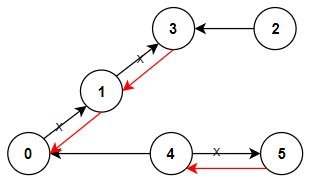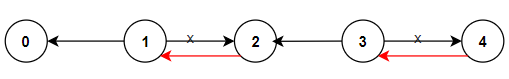# GeetCode Hub

There are `n` cities numbered from `0` to `n - 1` and `n - 1` roads such that there is only one way to travel between two different cities (this network form a tree). Last year, The ministry of transport decided to orient the roads in one direction because they are too narrow.

Roads are represented by `connections` where `connections[i] = [ai, bi]` represents a road from city `ai` to city `bi`.

This year, there will be a big event in the capital (city `0`), and many people want to travel to this city.

Your task consists of reorienting some roads such that each city can visit the city `0`. Return the minimum number of edges changed.

It's guaranteed that each city can reach city `0` after reorder.

Example 1:```Input: n = 6, connections = [[0,1],[1,3],[2,3],[4,0],[4,5]]
Output: 3
Explanation: Change the direction of edges show in red such that each node can reach the node 0 (capital).
```

Example 2:```Input: n = 5, connections = [[1,0],[1,2],[3,2],[3,4]]
Output: 2
Explanation: Change the direction of edges show in red such that each node can reach the node 0 (capital).
```

Example 3:

```Input: n = 3, connections = [[1,0],[2,0]]
Output: 0
```

Constraints:

• `2 <= n <= 5 * 104`
• `connections.length == n - 1`
• `connections[i].length == 2`
• `0 <= ai, bi <= n - 1`
• `ai != bi`

class Solution { public int minReorder(int n, int[][] connections) { } }